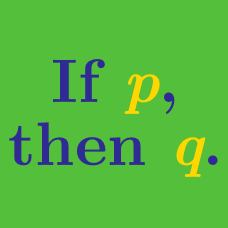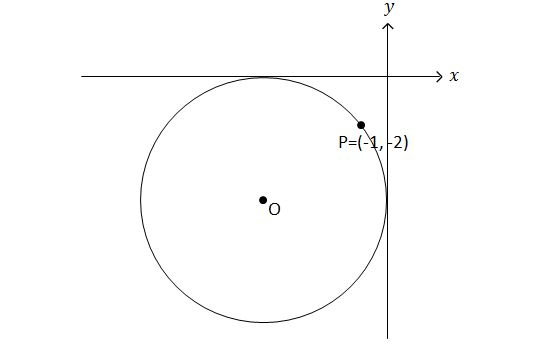Everyday Math

# Reasoning - Problem Solving

How many triples of positive integers $(a, b, c)$ are there such that $\begin{array}{c}&1\le \frac{a^2 + b^2}{c}\le 3, &a\le 5, &b\le 5, &c\le 5? \end{array}$

(A) $\ \ 12$
(B) $\ \ 17$
(C) $\ \ 19$
(D) $\ \ 21$
(E) $\ \ 34$

$\text{Pick-}3$ is a lottery in which three numbers are drawn from a larger pool of numbers $1$ through $x,$ with the order in which they are drawn irrelevant. If you want the odds of hitting the jackpot to be between $\frac{1}{2000}$ and $\frac{1}{3000},$ which of the following numbers is eligible for $x?$

$\begin{array}{r r l} & \text{I.} & 24\\ & \text{II.} & 26\\ & \text{III.} & 28\\ \end{array}$

(A)$\ \$ I only
(B)$\ \$ II only
(C)$\ \$ III only
(D)$\ \$ I and II only
(E)$\ \$ II and III only

If he does not trust me, he will not see me again.
If he does not see me again, I cannot work for him any more.
If I cannot work for him any more, I will have to look for another job.

Given the above $3$ statements, which of the following statements must be true?

$\begin{array}{r r l} & \text{I.} & \text{If I can keep working for him, then he trusts me.}\\ & \text{II.} & \text{If he sees me again, I do not have to look for another job.}\\ & \text{III.} & \text{If I have to look for another job, then he does not trust me.}\\ \end{array}$

(A)$\ \$ I only
(B)$\ \$ I and II only
(C)$\ \$ I and III only
(D)$\ \$ II and III only
(E)$\ \$ I, II and III

John leaves Chicago bound for New York at $5$ a.m. He drives at an average speed of $60$ miles per hour and arrives at a rest area to take a breakfast for half an hour. He leaves the rest area at $8$ a.m. and drives $200$ miles before taking one-hour break at another rest area. At $11$ a.m. John leaves the second area and drives the remaining $450$ miles straight on, taking only a $10$-minute break at the third rest area, until he arrives at New York at $6$ p.m. What is John's average speed between Chicago and the second rest area (including the first rest time where is speed is $0$ miles per hour in the calculation)?

(A) $\ \ 54 \text{ mph}$
(B) $\ \ 60 \text{ mph}$
(C) $\ \ 64 \text{ mph}$
(D) $\ \ 70 \text{ mph}$
(E) $\ \ 80 \text{ mph}$In the figure above, circle $O$ is tangent to both the $x$ and $y$ axes. If $P=(-1, -2)$ is a point on the circle, which of the following points is NOT on circle $O?$

$\begin{array}{r r l} & \text{I.} & (-1.5, -8.5)\\ & \text{II.} & (-5, 0)\\ & \text{III.} & (-8, -9)\\\ \end{array}$

(A)$\ \$ I only
(B)$\ \$ II only
(C)$\ \$ III only
(D)$\ \$ II and III only
(E)$\ \$ I, II, and III

×